# 轩田机器学习基石课程学习笔记11 — Linear Models for Classification

## 一、Linear Models for Binary Classification

s=w^Tx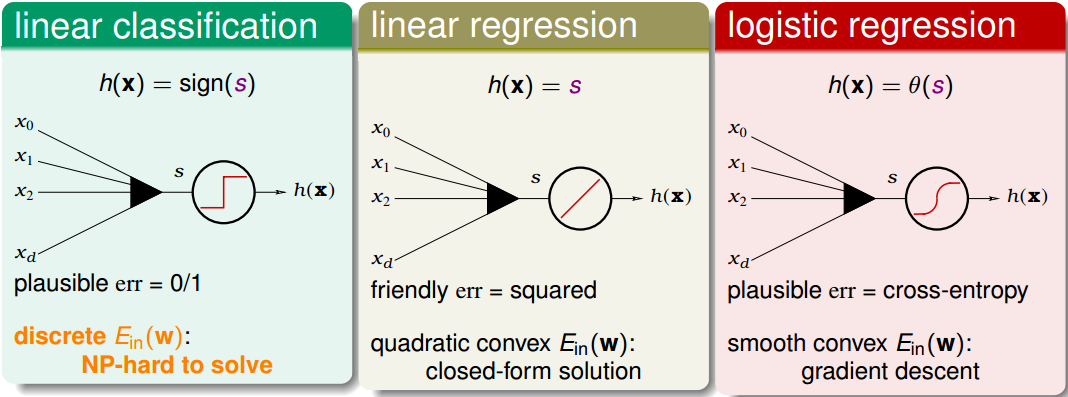err_{0/1}(s,y)=|sign(s)\neq y|=|sign(ys)\neq 1|

err_{SQR}(s,y)=(s-y)^2=(ys-1)^2

err_{CE}(s,y)=ln(1+exp(-ys))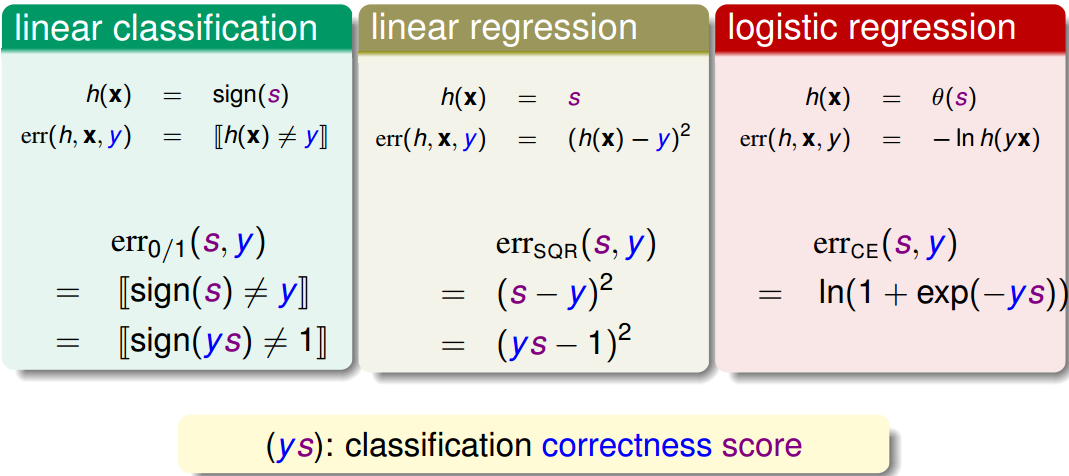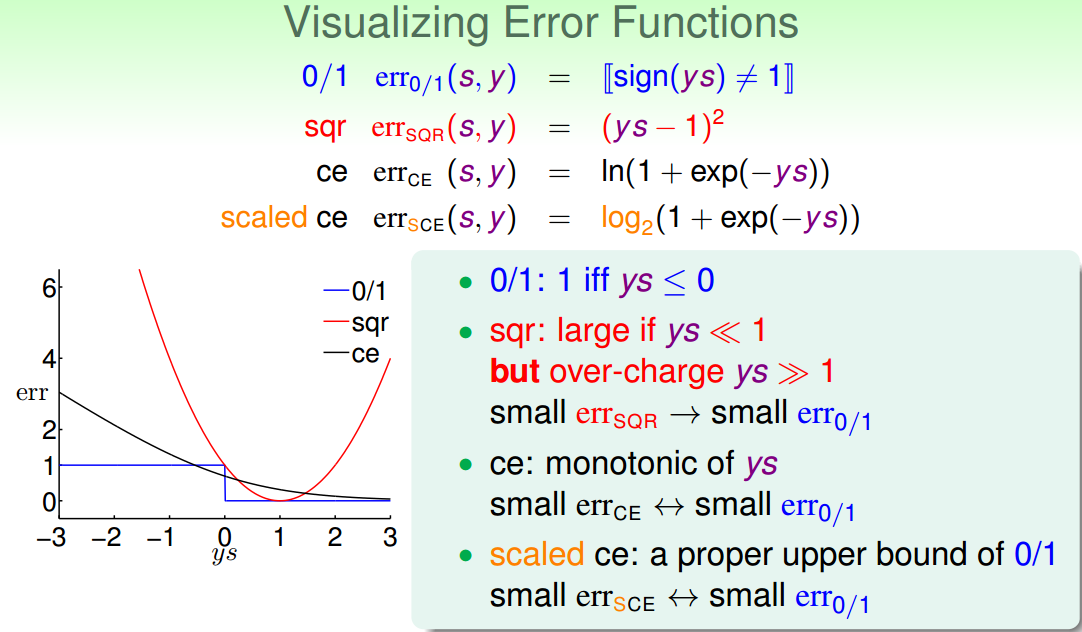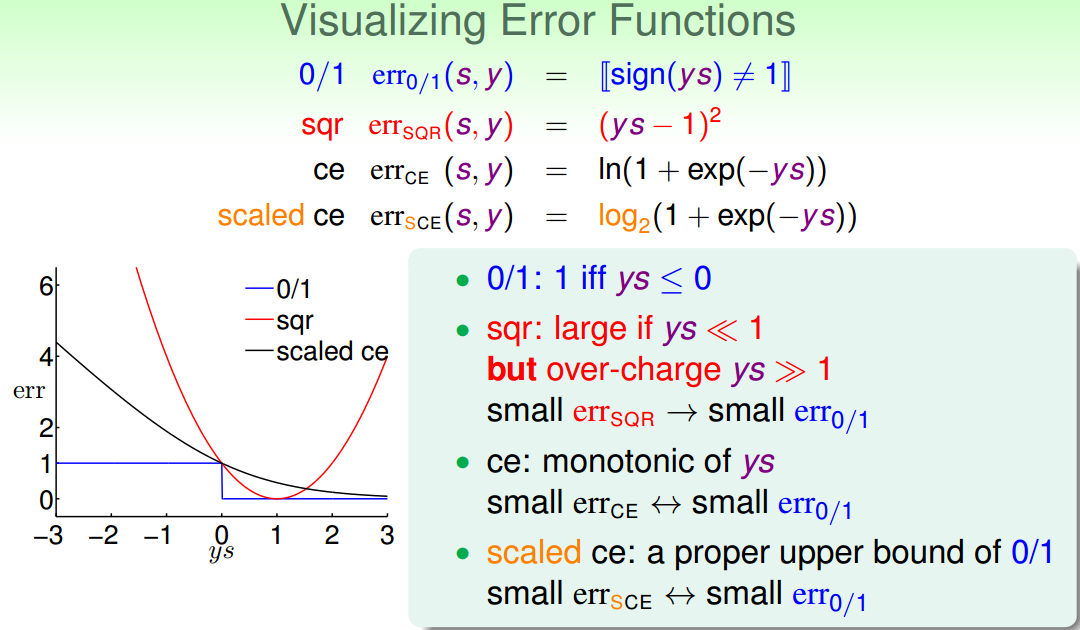err_{0/1}(s,y)\leq err_{SCE}(s,y)=\frac1{ln2}err_{CE}(s,y)

E_{in}^{0/1}(w)\leq E_{in}^{SCE}(w)=\frac1{ln2}E_{in}^{CE}(w)

E_{out}^{0/1}(w)\leq E_{out}^{SCE}(w)=\frac1{ln2}E_{out}^{CE}(w)

E_{out}^{0/1}(w)\leq E_{in}^{0/1}(w)+\Omega^{0/1}\leq \frac1{ln2}E_{in}^{CE}(w)+\Omega^{0/1}

E_{out}^{0/1}(w)\leq \frac1{ln2}E_{out}^{CE}(w)\leq \frac1{ln2}E_{in}^{CE}(w)+\frac1{ln2}\Omega^{CE}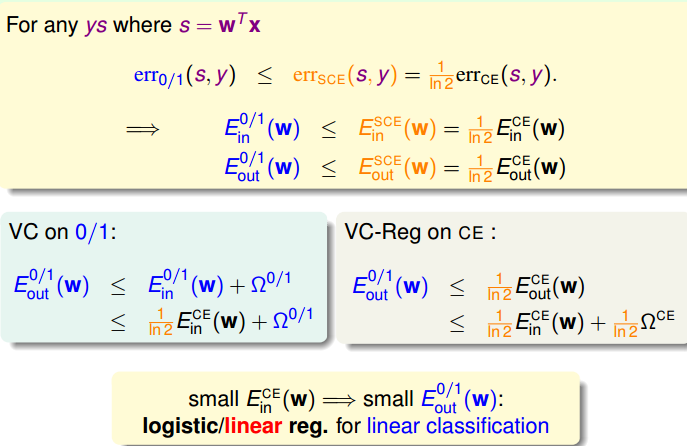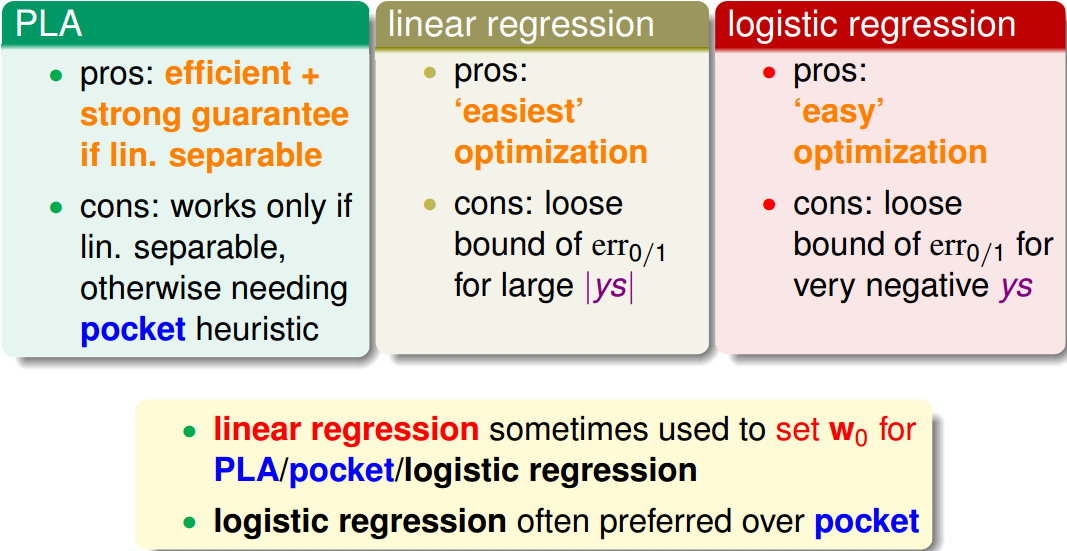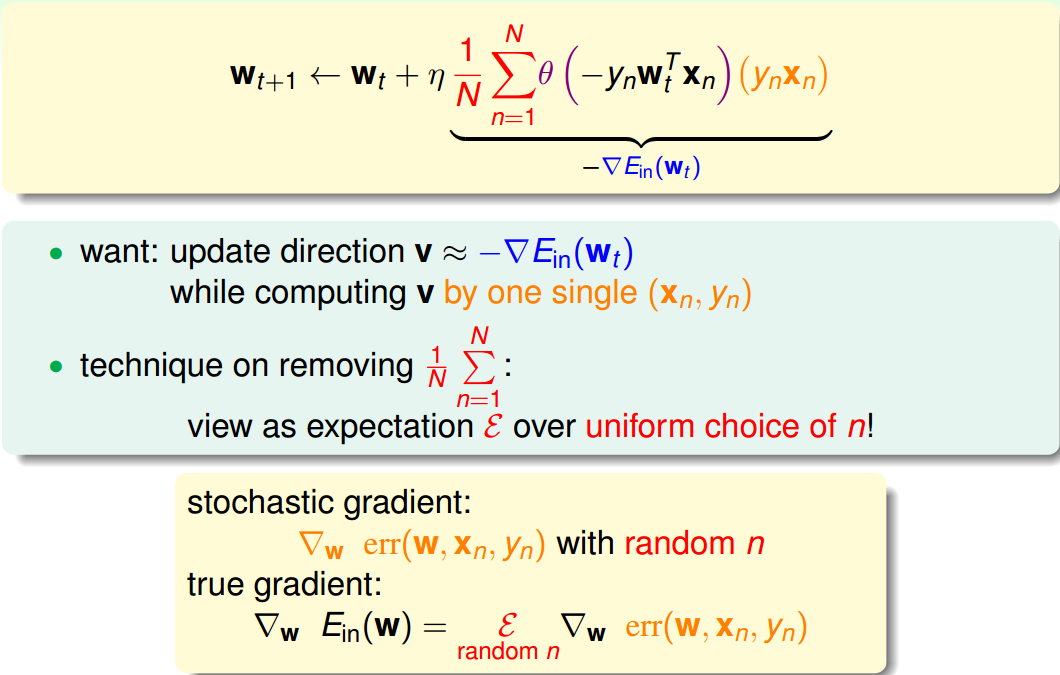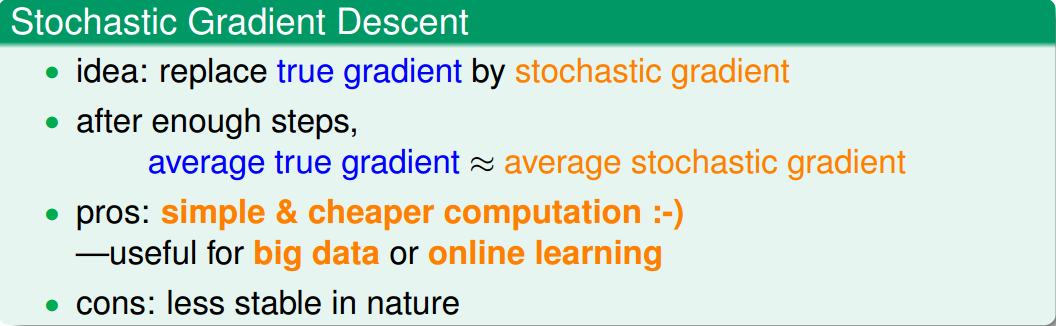w_{t+1}\leftarrow w_t+\eta\theta(-y_nw_t^Tx_n)(y_nx_n)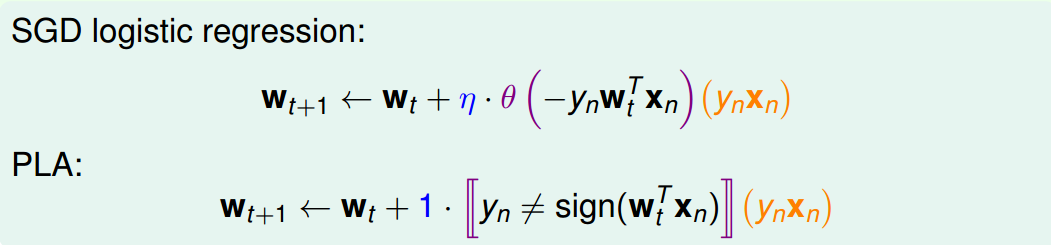## 三、Multiclass via Logistic Regression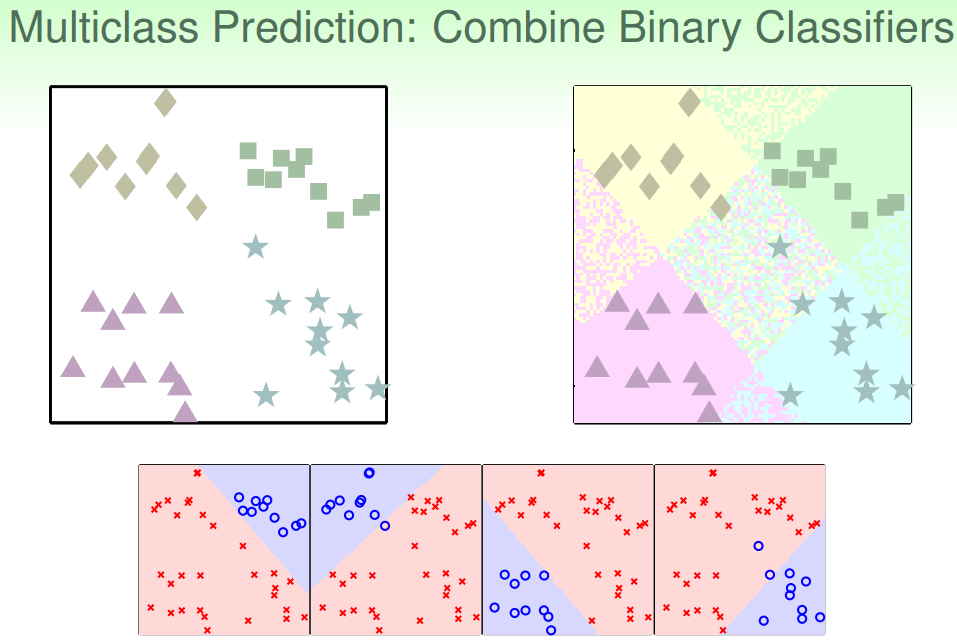soft classification的处理过程和之前类似，同样是分别令某类为正，其他三类为负，不同的是得到的是概率值，而不是{-1，+1}。最后得到某点分别属于四类的概率，取最大概率对应的哪一个类别就好。效果如下图所示：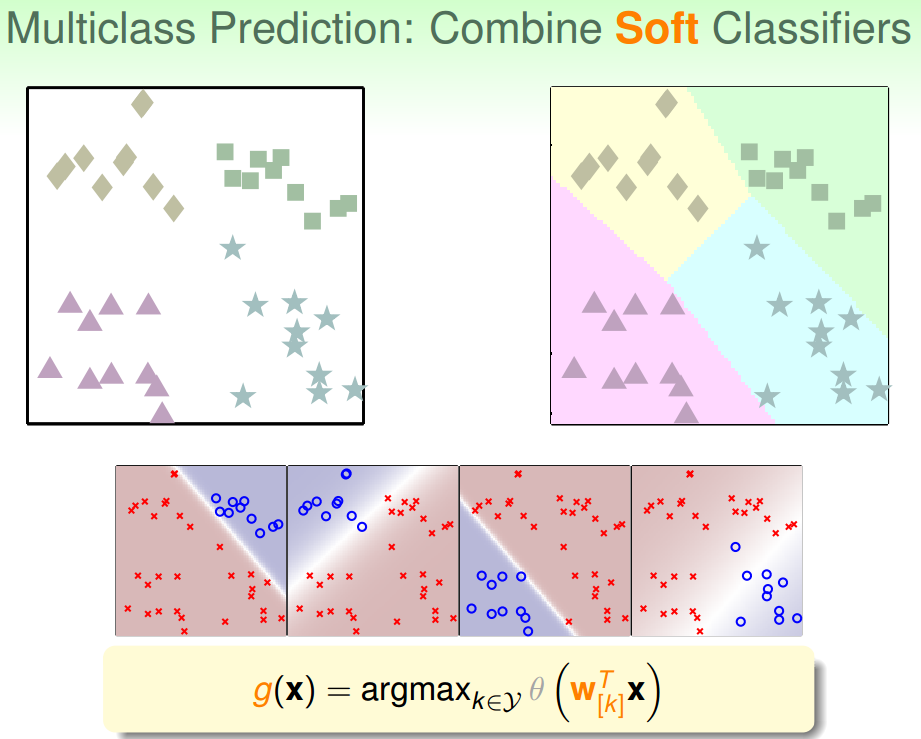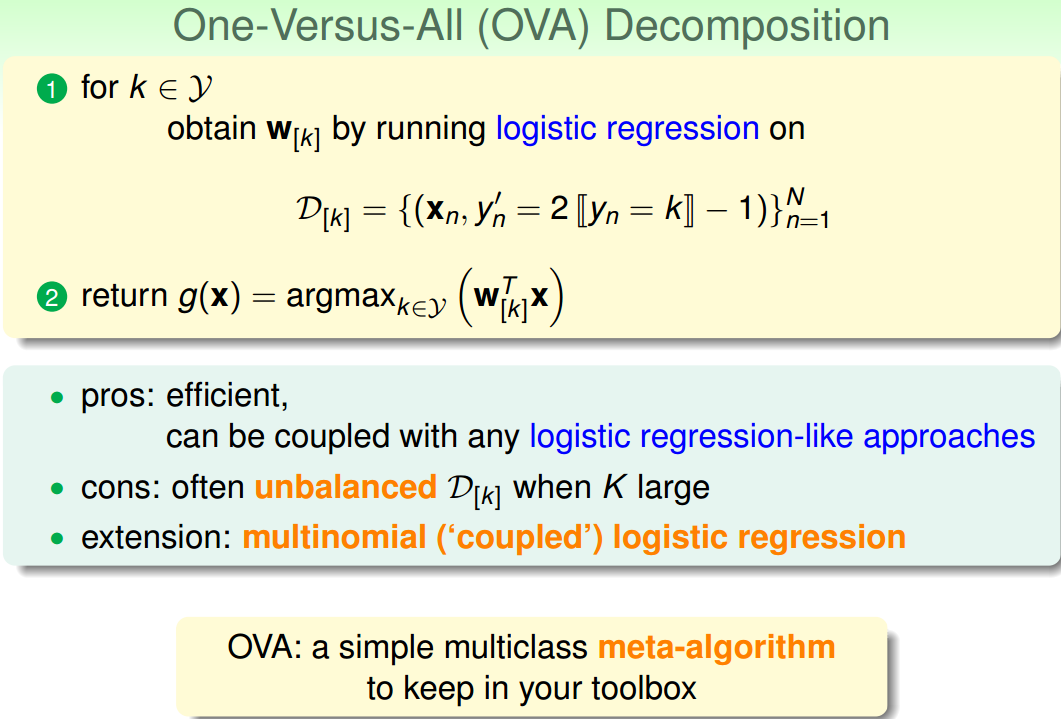## 四、Multiclass via Binary Classification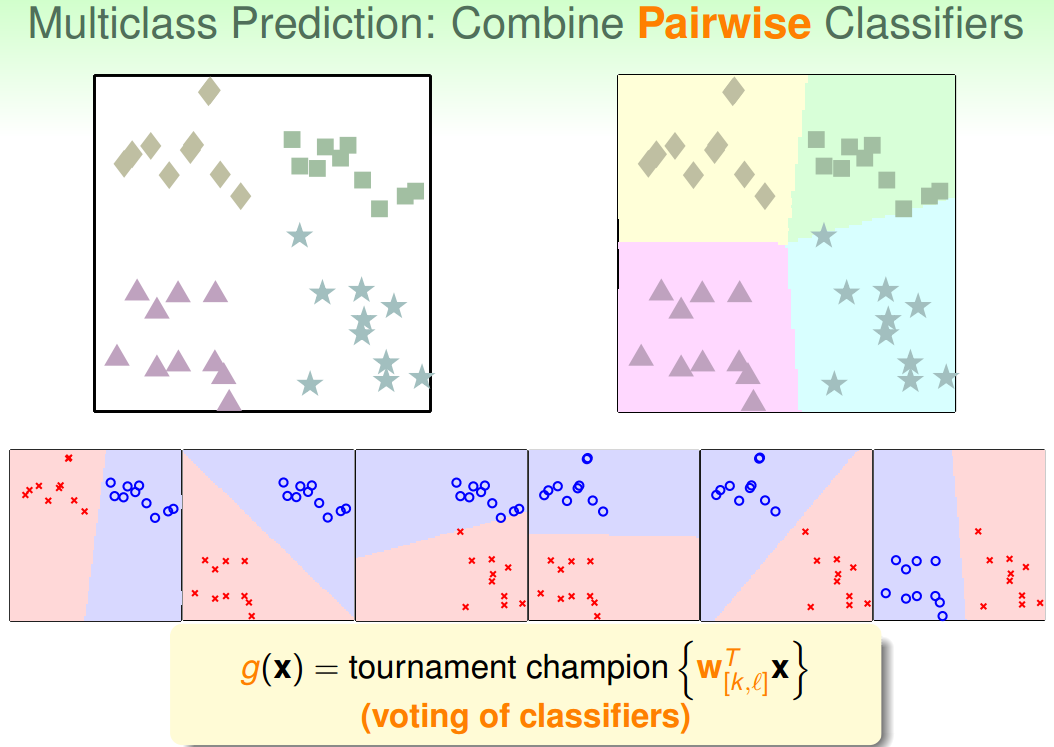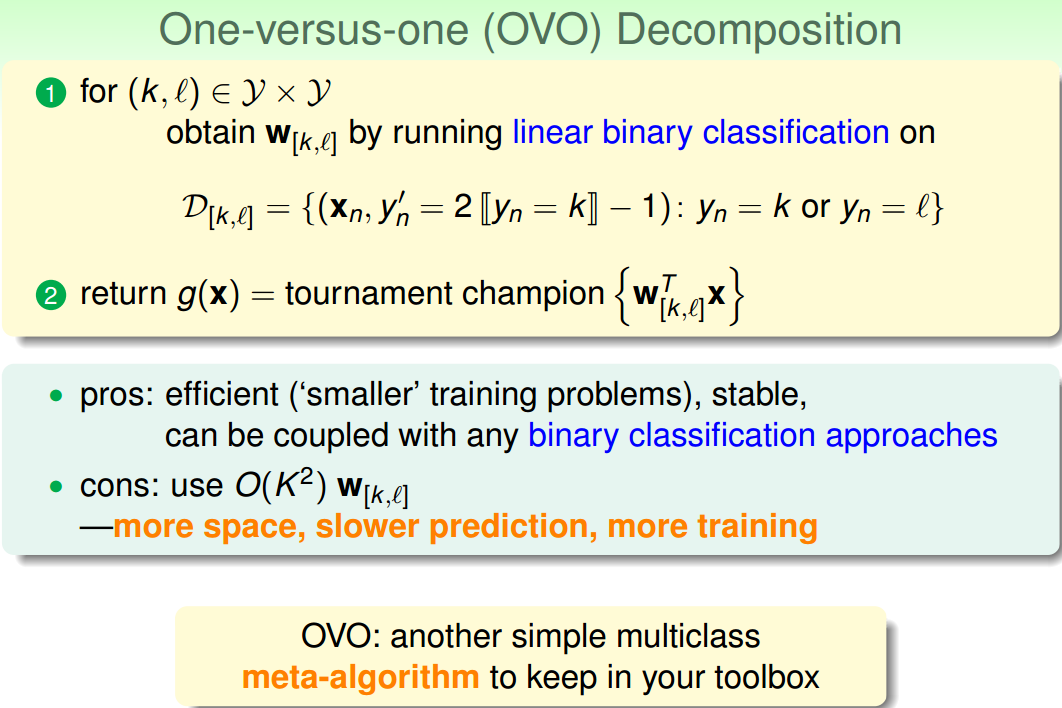## 五、总结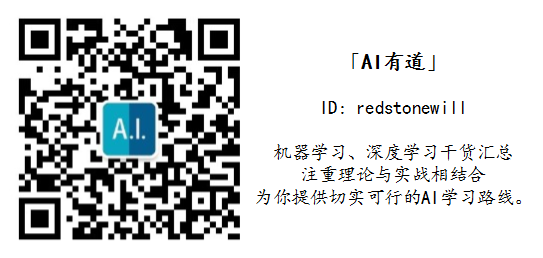### 评论 1

1. jiaqi一中 第一段 “逻辑回归模型的hypothesis为h(x)=\theta(s)h(x)=θ(s)，取值范围为(-1,1)之间 ” 应该是（0，1）之间吧回复

### 觉得文章有用就打赏一下文章作者

#### 支付宝扫一扫打赏#### 微信扫一扫打赏